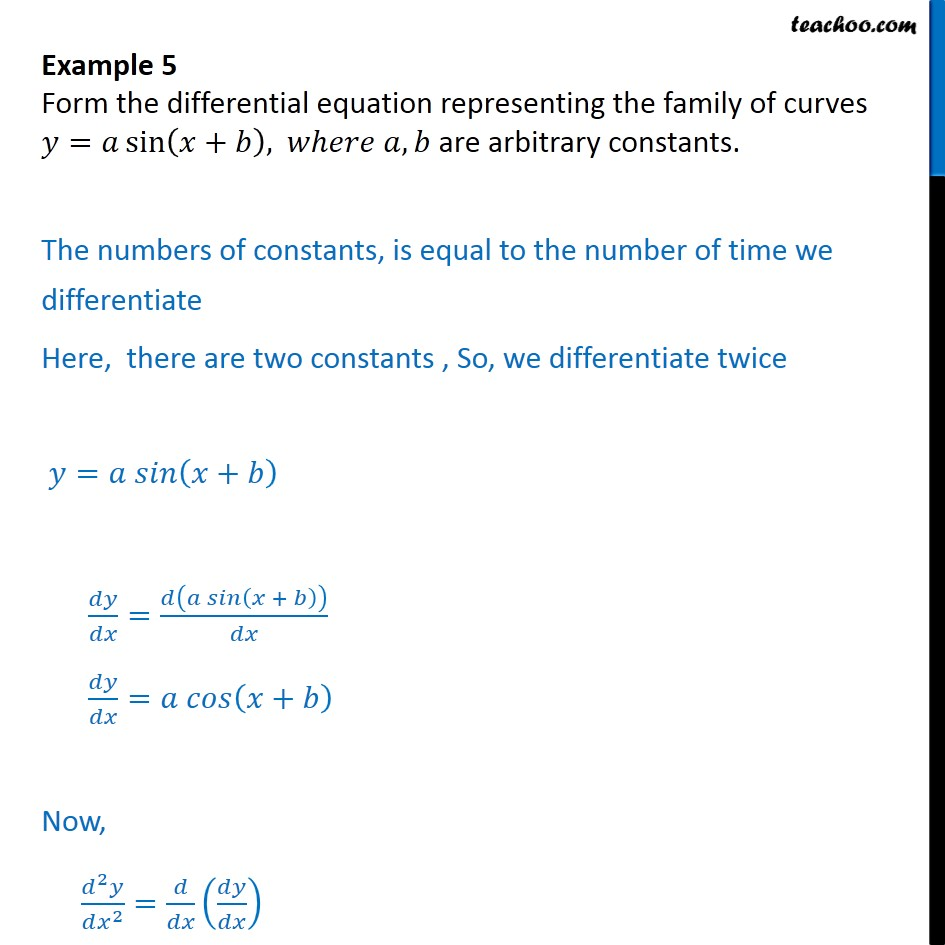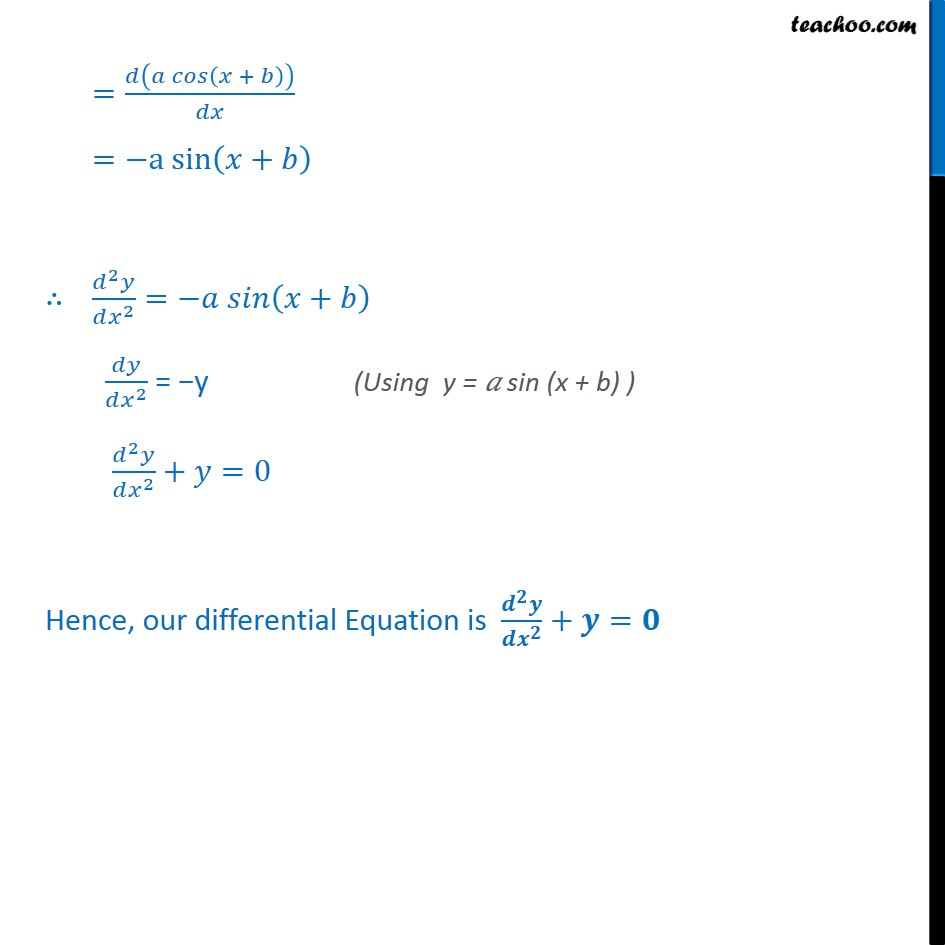Examples

Chapter 9 Class 12 Differential Equations
Serial order wiseLearn in your speed, with individual attention - Teachoo Maths 1-on-1 Class

### Transcript

Question 2 Form the differential equation representing the family of curves = sin + , , are arbitrary constants. The numbers of constants, is equal to the number of time we differentiate Here, there are two constants , So, we differentiate twice = + = + = + Now, 2 2 = = + = a sin + 2 2 = + 2 = y 2 2 + =0 Hence, our differential Equation is + =